## How to average every 5 rows or columns in Excel?

In Excel, have you ever tried to average every 5 rows or columns, that is to say, you need to do these operations: =average (A1:A5), =average(A6:A10), =average(A11:A15),…of course, you can apply the Average function to get the average of every 5 cells every time, but, if there are hundreds and thousands cells in your list, this will be tedious. Today, I will talk about some formulas to solve this job quickly and time-saving.

Average every 5 rows or columns with formulas

#### Average every 5 rows or columns with formulas

The following formula will help you to calculate the average of every 5 rows in the worksheet, please do as follows:

1. Enter this formula into a blank cell: =AVERAGE(OFFSET(\$A\$2,(ROW()-ROW(\$C\$2))*5,,5,)) (A2 is the start value that you want to average from, and C2 is the cell that you put this formula, the number 5 indicates every 5 rows you want to average), and then press Enter key to get the result, see screenshot: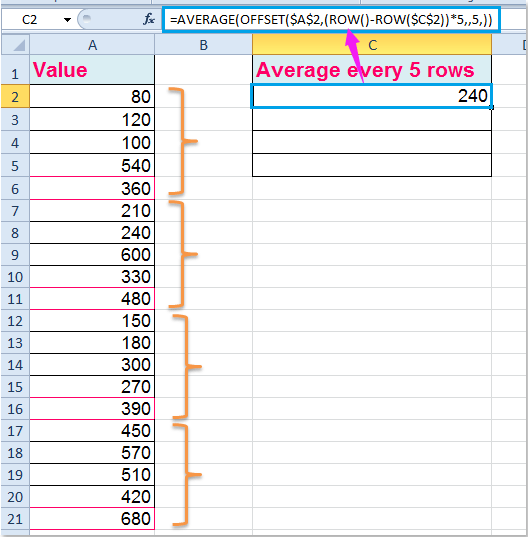2. Then select this cell and drag the fill handle down over to the cells until the error value displayed. See screenshot: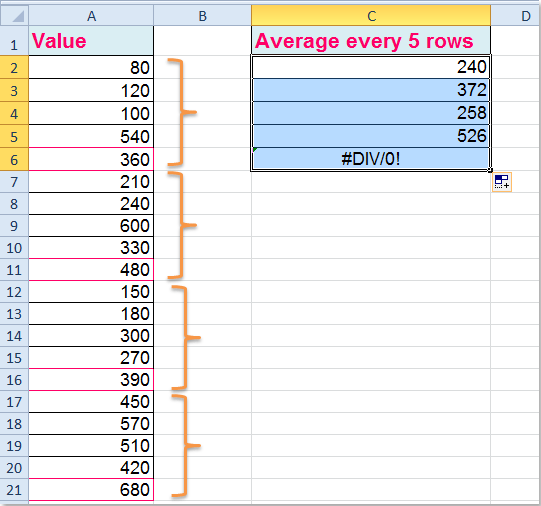Note: If you want to average every 5 columns in a row, you can apply this formula: =AVERAGE(OFFSET(\$A\$1,,(COLUMNS(\$A\$3:A3)-1)*5,,5)) (A1 is the start value that you want to average from, and A3 is the cell where you put this formula, the number 5 indicates every 5 columns you want to average), and then drag the fill handle to right side until the error value appeared, see screenshot: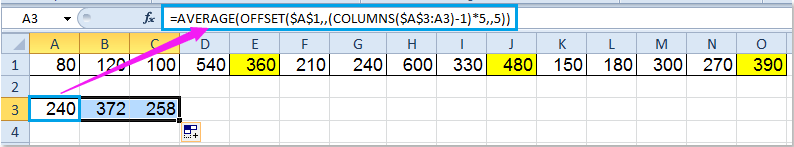#### Average every 5 rows or columns with Kutools for Excel

Kutools for Excel's Insert Page Breaks Every Row can help you to insert some page breaks for every n rows, and then average every n rows by applying the Paging Subtotals feature.

 : with more than 300 handy Excel add-ins, free to try with no limitation in 30 days.

After installing Kutools for Excel, please do as this:

1. Click Kutools Plus > Printing > Insert Page Break Every Row, see screenshot: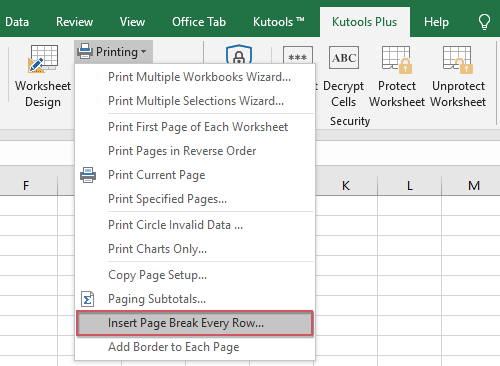2. In the Insert Page Break Every Row dialog box, specify the row number which you want to insert page breaks between, and the page breaks are inserted every 5 rows as following screenshot shown: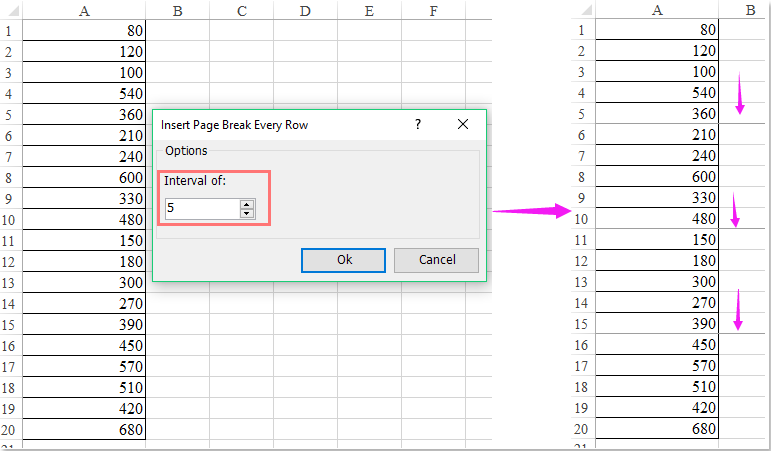3. And then click Kutools Plus > PrintingPaging Subtotals, see screenshot: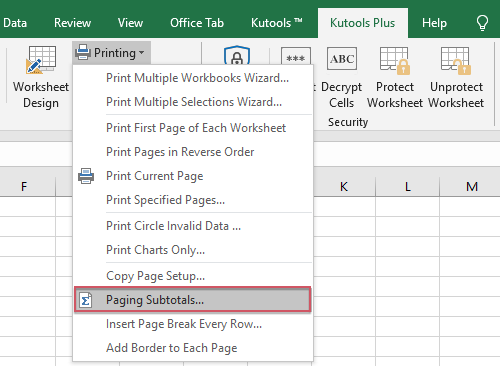4. In the Paging Subtotals dialog box, check the column header that you want to do some calculations, and then choose the function Average as you need, see screenshot: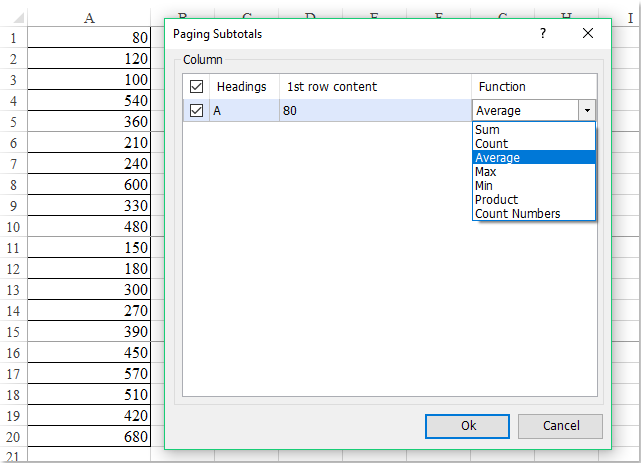5. And then, the average has been calculated every 5 rows, see screenshot: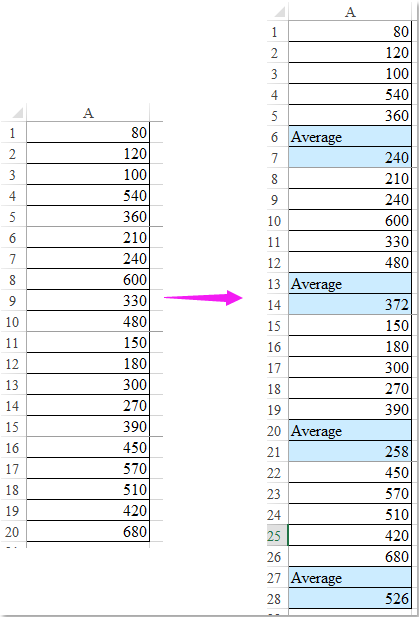#### Demo: Sum / Average every 5 rows or columns with Kutools for Excel

Kutools for Excel: with more than 300 handy Excel add-ins, free to try with no limitation in 30 days.

Related articles:

How to average last 5 values of a column as new numbers entering?

How to average top or bottom 3 values in Excel?

### Best Office Productivity Tools

 Popular Features: Find, Highlight or Identify Duplicates   |  Delete Blank Rows   |  Combine Columns or Cells without Losing Data   |   Round without Formula ... Super Lookup: Multiple Criteria VLookup  |   Multiple Value VLookup  |   VLookup Across Multiple Sheets   |   Fuzzy Lookup .... Advanced Drop-down List: Quickly Create Drop Down List   |  Dependent Drop Down List   |  Multi-select Drop Down List .... Column Manager: Add a Specific Number of Columns  |  Move Columns  |  Toggle Visibility Status of Hidden Columns  |  Compare Ranges & Columns ... Featured Features: Grid Focus   |  Design View   |   Big Formula Bar   |  Workbook & Sheet Manager   |  Resource Library (Auto Text)   |  Date Picker   |  Combine Worksheets   |  Encrypt/Decrypt Cells   |  Send Emails by List   |  Super Filter   |   Special Filter (filter bold/italic/strikethrough...) ... Top 15 Toolsets:  12 Text Tools (Add Text, Remove Characters, ...)   |   50+ Chart Types (Gantt Chart, ...)   |   40+ Practical Formulas (Calculate age based on birthday, ...)   |   19 Insertion Tools (Insert QR Code, Insert Picture from Path, ...)   |   12 Conversion Tools (Numbers to Words, Currency Conversion, ...)   |   7 Merge & Split Tools (Advanced Combine Rows, Split Cells, ...)   |   ... and more

Supercharge Your Excel Skills with Kutools for Excel, and Experience Efficiency Like Never Before. Kutools for Excel Offers Over 300 Advanced Features to Boost Productivity and Save Time.  Click Here to Get The Feature You Need The Most...#### Office Tab Brings Tabbed interface to Office, and Make Your Work Much Easier

• Enable tabbed editing and reading in Word, Excel, PowerPoint, Publisher, Access, Visio and Project.
• Open and create multiple documents in new tabs of the same window, rather than in new windows.
• Increases your productivity by 50%, and reduces hundreds of mouse clicks for you every day!No ratings yet. Be the first to rate!
This comment was minimized by the moderator on the site
not working at all
This comment was minimized by the moderator on the site
This comment was minimized by the moderator on the site
Thank you so much!
This comment was minimized by the moderator on the site
The goal is to capture 5 rows of adjacent data items, in the same column, in groups of 5. The REFERENCE cell is A2. When the FORMULA is typed in, for example, cell C2, meaning Column C Row 2, the numeric result of ROW() = 2. Therefore, in order to begin the counter AT the starting point REFERENCE cell selected, in this site's example A2, the operator for OFFSET of rows needs to 0. 0 means Start Here at the REFERENCE cell. ROW() returns the row number as an integer. ROW () in the cell in which the FORMULA is typed, C2, returns the value 2: ROW()-2 = (2)-2 = 0. In order to count ahead 5 rows each time, *5 is added. Add parenthesis around the subtraction because of mathematical order of operations: (ROW()-2). When the FORUMULA is typed in row C2, (ROW()-2)*5 = ((2)-2)*5 = (0)*5 =0. Copy this formula down into cell C3 and the result is (ROW()-2*5=((3)-2)*5 = (1)*5 = 5 which initiates the OFFSET function of the FORMULA to begin at 5 rows below cell A2 : A(2+5) = A7. Copy this formula down into cell C4, the result is (ROW()-2*5=((4)-2)*5 = (2)*5 = 10 which causes OFFSET of the FORMULA to begin at 10 rows below cell A2 : A(2+10) = A12. In order to capture 5 items of data, the number in [height] = 5. The complete FORMULA typed in cell C2 is =AVERAGE(OFFSET(\$A\$2,(ROW()-2)*5,0,5,1)). The function AVERAGE can be replaced with SUM, STDEV, etc.
This comment was minimized by the moderator on the site
how to find average between two consecutive zero value in coloumn .
This comment was minimized by the moderator on the site
Thanks heaps for this information. Very helpful for working with my data set.
This comment was minimized by the moderator on the site
This worked so well, i'm a little skeptical because I am dealing with huge chunks of data and its difficult to verify if i'm getting correct values
This comment was minimized by the moderator on the site
Thanks a lot extend office you saved my lot of time...Will definitely love to contribute one day when i become rich.
There are no comments posted here yet